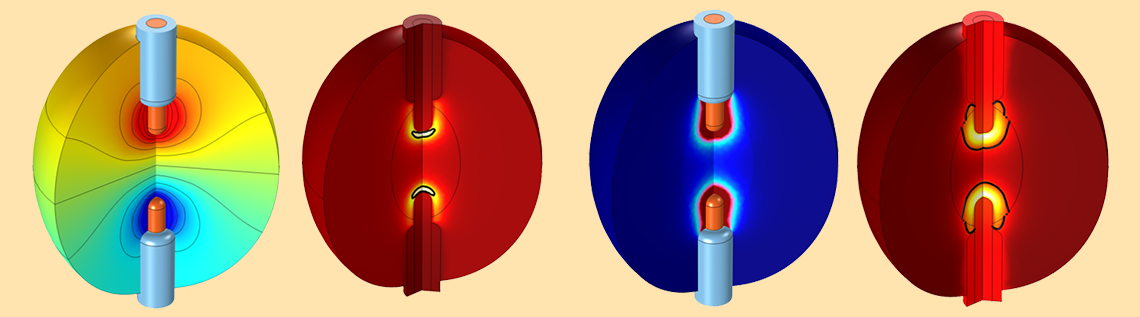# 生物组织射频消融技术的仿真研究

2016年 1月 20日

### 什么是射频组织消融？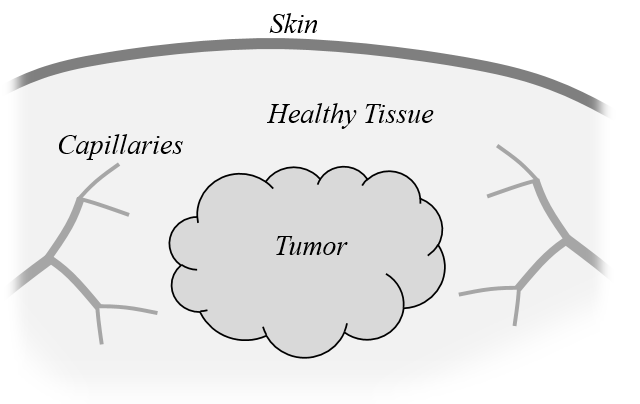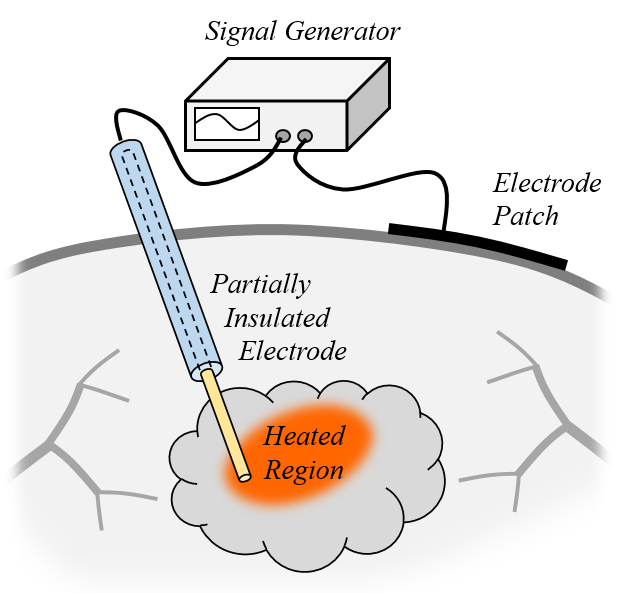### 分析焦耳热和电流

“平均”的人体组织 0.5 65 1 0.5 + j 0.0003

\nabla \cdot \left[ (\sigma+j\omega \epsilon_0 \epsilon_r) \nabla V \right] = 0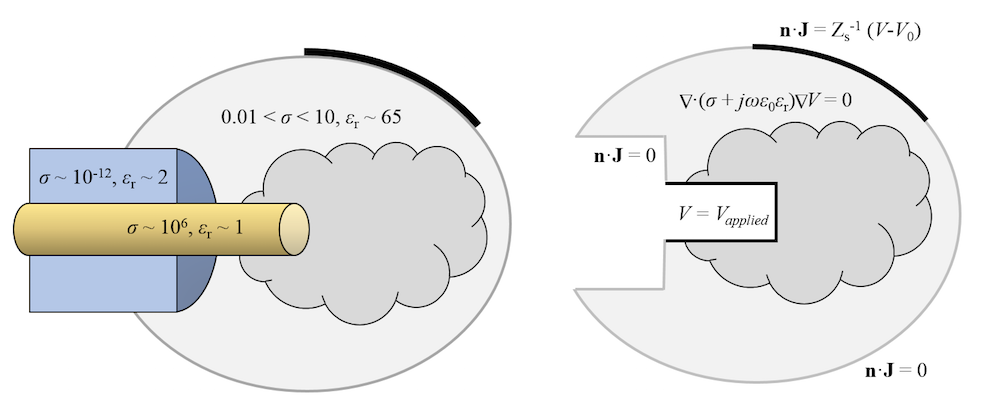### 计算人体组织中的温度升高

\rho C_p \frac{\partial T}{ \partial t}-\nabla \cdot k \nabla T = \frac{_1}{^2}\Re(\mathbf{J^*}\cdot \mathbf{E})+\rho_b C_{p,b} \omega_b(T_b-T) + Q_{met}

\mathbf{n} \cdot k \nabla T = h (T_{ext}-T)

• 特定时间内的温度阈值分析：如果在特定时间内，受热组织超过了特定的损伤温度（例如 50 秒之内超过 50°C），或者峰值温度瞬间超过了可致坏死的温度（例如 100 °C），人们就认为组织遭受了不可逆的损伤。人们还基于损伤温度和时间来计算组织的损伤比例。举例来说，50℃ 高温持续 25 秒后，将导致 50％ 的损伤。
• 能量吸收分析：采用“频域因子”和“活化能”作为被研究对象的特性，使用 Arrhenius 方程来计算受损组织所占的比例。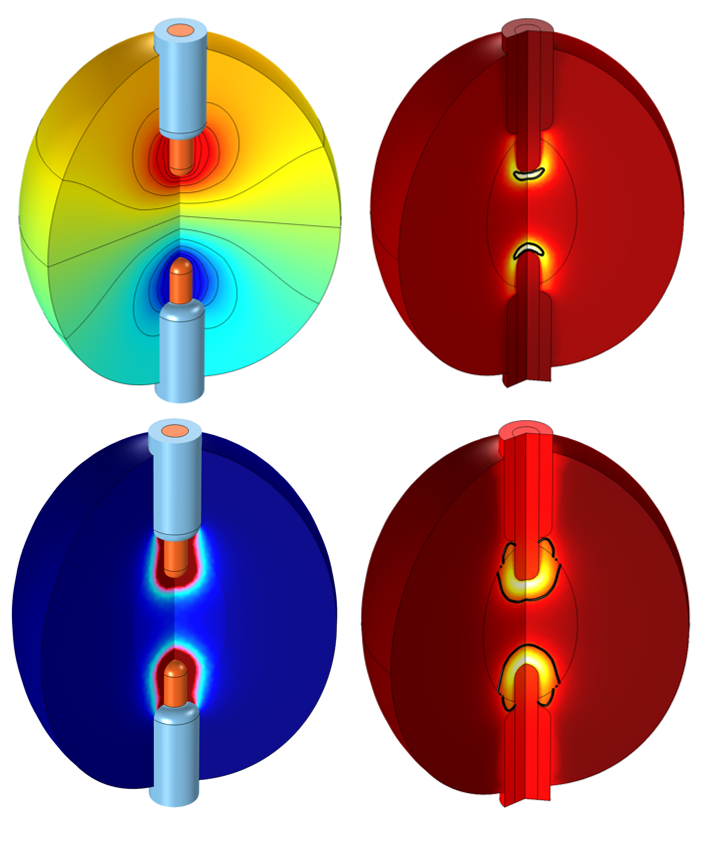### 探索更多关于射频组织消融建模的资源

#### 评论 (1)

##### 留言2019-04-09